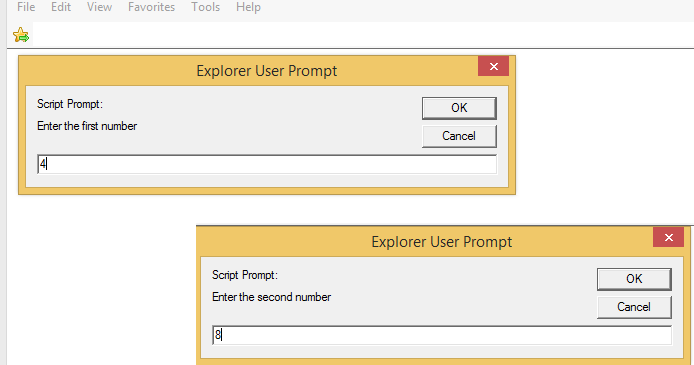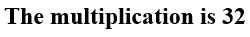### Mathematical Operations

Appying a basic mathemeatical operation (+,-,*,/, and reminder after division %) in JavaScript is very strainforward.
A JavaScript program shown here illustrates how to multiply two numbers entered by the user:

```	<!DOCTTYPE html>
<!--Example 1: Promt2.html -->
<html>
<head>
<title> Prompt the user to enter two entries </title>
<script >
<!--

//read the first number as a string
firstentry = window.prompt('Enter the first number');
//read the second number as a string
secondentry = window.prompt('Enter the second number');
//convert strings to numbers
number1=parseInt(firstentry);
number2=parseInt(secondentry);
mult = number1* number2; //multiplying the two numbers
//show he result
document.writeln('<h2> The multiplication is ' + mult + '</h2>');
// -->
</script>
</head>
<body></body>
</html>
```
Note that any entry is first interpreted as a string, and if it's a number it needs to be converted into a number as shown in this program.
First, the user is prompted to enter two numbers:Then multiplicatiopn is shown as under:For more details, please contact me here.
Date of last modification: March 26, 2019.